# 1720 Transforming Puzzle-A-Day’s Wizard’s Hat

### Today’s Puzzle:

I recently saw a fun-to-solve puzzle from Puzzle a Day on Twitter:

The puzzle got me thinking, what would happen if the numbers weren’t from 1 to 10, but were from 0 to 9 instead? Would it change the puzzle a little or a lot?It actually changes the puzzle quite a bit. There are a lot more solutions than for the Puzzle a Day puzzle! And I don’t mean just switching the red line with the green line and/or switching the purple circles at the bottom. How many solutions can you find?

### Factors of 1720:

• 1720 is a composite number.
• Prime factorization: 1720 = 2 × 2 × 2 × 5 × 43, which can be written 1720 = 2³ × 5 × 43.
• 1720 has at least one exponent greater than 1 in its prime factorization so √1720 can be simplified. Taking the factor pair from the factor pair table below with the largest square number factor, we get √1720 = (√4)(√430) = 2√430.
• The exponents in the prime factorization are 3,1 and 1. Adding one to each exponent and multiplying we get (3 + 1)(1 + 1) (1 + 1) = 4 × 2 × 2 = 16. Therefore 1720 has exactly 16 factors.
• The factors of 1720 are outlined with their factor pair partners in the graphic below.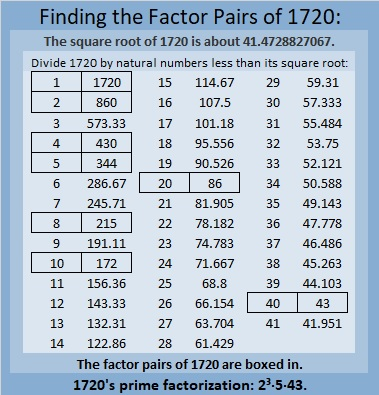### More About the Number 1720:

1720 is the difference of two squares in FOUR different ways:
431² – 429² = 1720,
217² – 213² = 1720,
91² – 81² = 1720, and
53² – 33² = 1720.

# 1717 Factor Fits for Your Valentine

### Today’s Puzzle:

Solve this Valentine-themed Factor Fits puzzle with both logic and heart!### Factors of 1717:

• 1717 is a composite number.
• Prime factorization: 1717 = 17 × 101.
• 1717 has no exponents greater than 1 in its prime factorization, so √1717 cannot be simplified.
• The exponents in the prime factorization are 1 and 1. Adding one to each exponent and multiplying we get (1 + 1)(1 + 1) = 2 × 2 = 4. Therefore 1717 has exactly 4 factors.
• The factors of 1717 are outlined with their factor pair partners in the graphic below.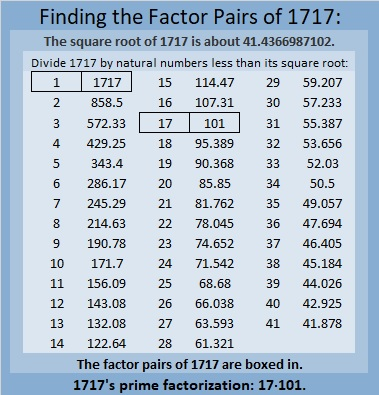### More About the Number 1717:

1717 is the sum of two squares two different ways:
41² + 6² = 1717, and
39² + 14² = 1717.

1717 is the hypotenuse of FOUR Pythagorean triples:
340 1683 1717, which is 17 times (20-99-101),
492 1645 1717, calculated from 2(41)(6), 41² – 6², 41² + 6²,
808 1515 1717, which is (8-15-17) times 101, and
1092 1325 1717, calculated from 2(39)(14), 39² – 14², 39² + 14².

# Two Factor Pairs of 1716 Make Sum-Difference!

### Today’s Puzzle:

How many factor pairs does 1716 have? Twelve. The factors in one of those factor pairs add up to 145 and the factors in a different one subtract to 145. If you can find those two factor pairs, then you can solve this puzzle!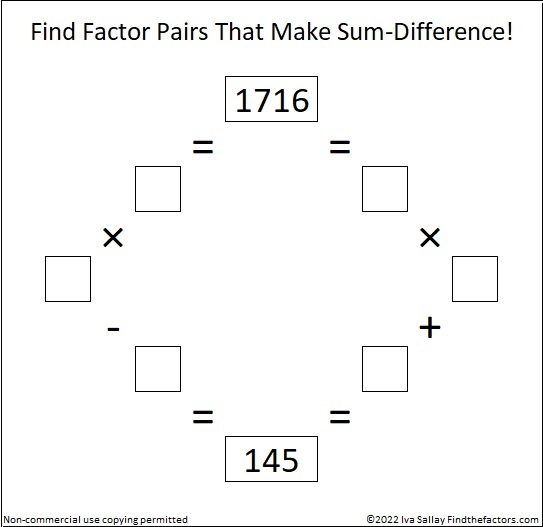Try to solve it all by yourself, but if you need some help, you can scroll down to learn more about the factors of 1716.

### A Factor Tree for 1716:

3 is a factor of 1716 because 1 + 7 + 1 + 6 = 15, a multiple of 3.
11 is a factor of 1716 because 1 – 7 + 1 – 6 = -11.

3 ·11 is 33. Here is one of MANY possible factor trees for 1716. This one uses the fact that 33 · 52 = 1716: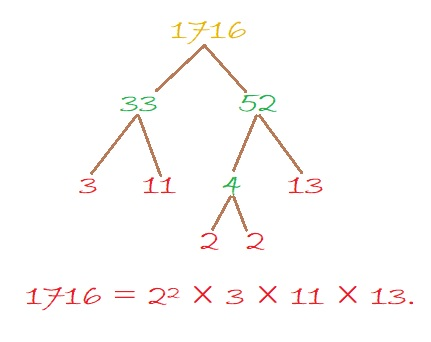### Factors of 1716:

• 1716 is a composite number.
• Prime factorization: 1716 = 2 × 2 × 3 × 11 × 13, which can be written 1716 = 2² × 3 × 11 × 13.
• 1716 has at least one exponent greater than 1 in its prime factorization so √1716 can be simplified. Taking the factor pair from the factor pair table below with the largest square number factor, we get √1716 = (√4)(√429) = 2√429.
• The exponents in the prime factorization are 2, 1, 1, and 1. Adding one to each exponent and multiplying we get (2 + 1)(1 + 1)(1 + 1)(1 + 1) = 3 × 2 × 2 × 2 = 24. Therefore 1716 has exactly 24 factors.
• The factors of 1716 are outlined with their factor pair partners in the graphic below.Here’s a chart of those same factor pairs but in reverse order with their sums and differences included.### More About the Number 1716:

1716 is the hypotenuse of a Pythagorean triple:
660-1584-1716, which is (5-12-13) times 132.

OEIS.org reminds us that 1716 is in the 6th and 7th columns of the 13th row of Pascal’s Triangle: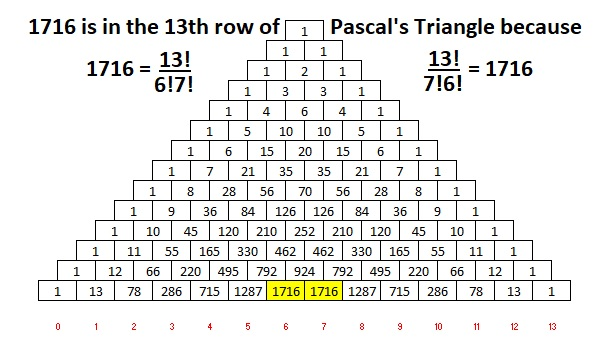# 1715 A Lot More of a Subtraction Distraction

### Today’s Puzzle:

Last time I published a puzzle with the last clue missing. Leaving out the first or the last clue only makes the puzzle slightly more difficult. What if I left out a clue more in the middle of the puzzle. I gave that some thought and designed today’s puzzle. I soon realized that I had to let you know that the 12 is one of the last eight boxes. There is only one solution. Can you find it?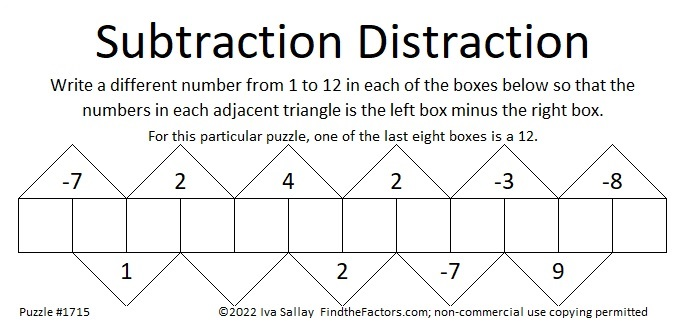I posted a solution video for it on Twitter:

### Factors of 1715:

• 1715 is a composite number.
• Prime factorization: 1715 = 5 × 7 × 7 × 7, which can be written 1715 = 5 × 7³.
• 1715 has at least one exponent greater than 1 in its prime factorization so √1715 can be simplified. Taking the factor pair from the factor pair table below with the largest square number factor, we get √1715 = (√49)(√35) = 7√35.
• The exponents in the prime factorization are 1 and 3. Adding one to each exponent and multiplying we get (1 + 1)(3 + 1) = 2 × 4 = 8. Therefore 1715 has exactly 8 factors.
• The factors of 1715 are outlined with their factor pairs in the graphic below.### More About the number 1715:

1715 = 1·7³·1·5. Thank you, OEIS.org for that fun fact!

1715 is the hypotenuse of a Pythagorean triple:
1029-1372-1715, which is (3-4-5) times 343.

1715 = 5·7³.
5·7º = 2² + 1².
5·7¹ cannot be written as the sum of two squares.
5·7² = 14² + 7².
5·7³ cannot be written as the sum of two squares.
5·7⁴ = 98² + 49².

What do you notice? What do you wonder?

# 1714 Will the Factors in This Puzzle Give You Fits?

### Today’s Puzzle:

12 and 24 have several common factors, but only one of them works in this puzzle. Will it be 2, 3, 4, 6, or 12?

What about 40 and 60’s common factors?

Don’t guess which factor to use. Start elsewhere in the puzzle where there’s only one possible common factor. Then use logic to eliminate some of the factor possibilities for 12, 24 and 40, 60. You will have to think, but it won’t be too difficult.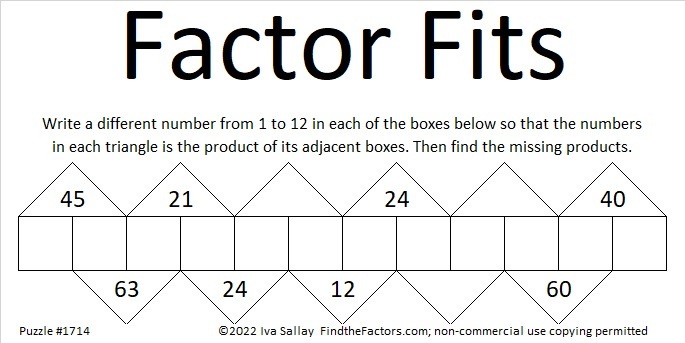### Factors of 1714:

• 1714 is a composite number.
• Prime factorization: 1714 = 2 × 857.
• 1714 has no exponents greater than 1 in its prime factorization, so √1714 cannot be simplified.
• The exponents in the prime factorization are 1 and 1. Adding one to each exponent and multiplying we get (1 + 1)(1 + 1) = 2 × 2 = 4. Therefore 1714 has exactly 4 factors.
• The factors of 1714 are outlined with their factor pair partners in the graphic below.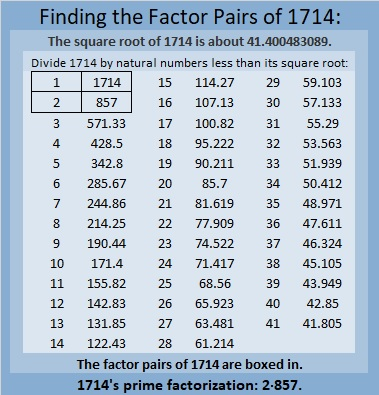### More About the Number 1714:

1714 is the sum of two squares:
33² + 25² = 1714.

1714 is the hypotenuse of a Pythagorean triple:
464-1650-1714, calculated from 33² – 25², 2(33)(25), 33² + 25².
It is also 2 times (232-825-857).

# 1713 A Little More of a Subtraction Distraction

### Today’s Puzzle:

It occurred to me that as long as the last box is neither 1 nor 12 that I could leave the clue above it blank. Can you still solve the puzzle?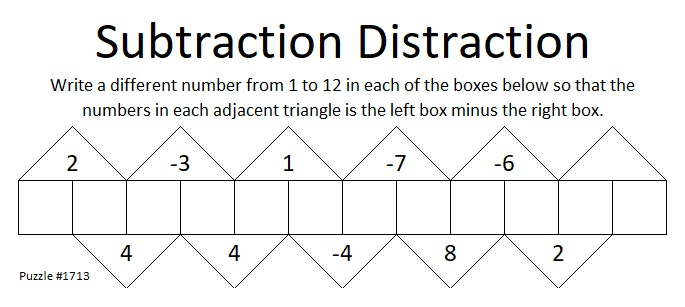### Factors of 1713:

• 1713 is a composite number.
• Prime factorization: 1713 = 3 × 571.
• 1713 has no exponents greater than 1 in its prime factorization, so √1713 cannot be simplified.
• The exponents in the prime factorization are 1 and 1. Adding one to each exponent and multiplying we get (1 + 1)(1 + 1) = 2 × 2 = 4. Therefore 1713 has exactly 4 factors.
• The factors of 1713 are outlined with their factor pair partners in the graphic below.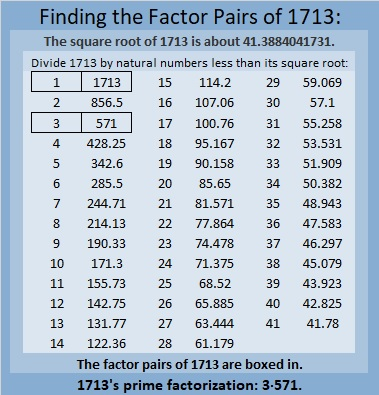### More About the Number 1713:

1713 is the difference of two squares in two different ways:
857² – 856² = 1713, and
287² – 284² = 1713.

1713 is the sum of two, three, and six consecutive numbers:
856 + 857 = 1713,
570 + 571 + 572 = 1713, and
283 + 284 + 285 + 286 + 287 + 288 = 1713.

Do you see any relationship between those two facts?

# 1712 Can You Make the Factors Fit?

### Today’s Puzzle:

This Factor Fits puzzle starts off fairly easy before it potentially might give you fits trying to place the rest of the factors. Are you game?### Factors of 1712:

• 1712 is a composite number.
• Prime factorization: 1712 = 2 × 2 × 2 × 2 × 107, which can be written 1712 = 2⁴ × 107.
• 1712 has at least one exponent greater than 1 in its prime factorization so √1712 can be simplified. Taking the factor pair from the factor pair table below with the largest square number factor, we get √1712 = (√16)(√107) = 4√107.
• The exponents in the prime factorization are 4 and 1. Adding one to each exponent and multiplying we get (4 + 1)(1 + 1) = 5 × 2 = 10. Therefore 1712 has exactly 10 factors.
• The factors of 1712 are outlined with their factor pair partners in the graphic below.### More About the Number 1712:

1712 is the difference of two squares in three different ways:
429² – 427² = 1712,
216² – 212² = 1712, and
111² – 103² = 1712.

# 1711 is a Triangular Number So The Taxman Wants His Share

### Today’s Puzzle:

Imagine this puzzle is made up of 58 envelopes, each containing the amount of money printed in bold type on its front. You can’t take any of the envelopes without the Taxman also taking a share. The Taxman will take EVERY available envelope that has a factor of the number you take on it. When you have taken all the cash you can, the Taxman gets ALL the leftover cash, and the game is over. You want the Taxman to get as little money as possible.

How much money is at stake? (58 × 59)/2 = 1711. That means 1711 is a triangular number, the sum of all the numbers from 1 to 58. Thus, the total amount of money you will be splitting with the Taxman is 1711.

To play this game, you can print the cards from this excel file: Taxman & 1537-1544. The factors of a number is printed in small type at the top of its card.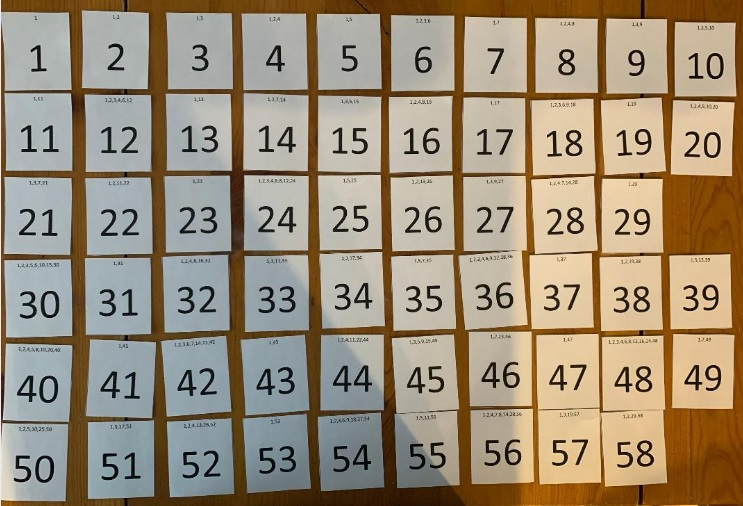Here below I show the order I selected the cards when I played. For example, I took 53, and all the Taxman got was 1. I took 49, and the only card left for the Taxman to take was 7, and so forth. The last card I could take was 42, and the Taxman got 21, but since I couldn’t take anymore cards, the Taxman also got 31, 34, 37, 41, 43, and 47.To win the game, you must get over half of the 1711 cash, but of course, you will want the Taxman to get much less than nearly half the money.

I didn’t want to make a long addition problem to find out how much I kept, so I came up with an easier strategy: I pushed the Taxman’s share to the side, and looked for ways to make 100 from my cards. (It is almost the 100th day of school, after all.) I found 9 ways to make 100, so I clearly kept more than half of the 1711.I added that final 53 + 50 + 39 + 36 in my head by thinking that if I took 3 away from 53 and gave it to the 36, it would be the same as 50 + 50 + 39 + 39, a fairly easy sum.

### Factors of 1711:

• 1711 is a composite number.
• Prime factorization: 1711 = 29 × 59.
• 1711 has no exponents greater than 1 in its prime factorization, so √1711 cannot be simplified.
• The exponents in the prime factorization are 1 and 1. Adding one to each exponent and multiplying we get (1 + 1)(1 + 1) = 2 × 2 = 4. Therefore 1711 has exactly 4 factors.
• The factors of 1711 are outlined with their factor pair partners in the graphic below.

### 1711 is a Shape-Shifting Number:

• 1711 is the 58th Triangular Number because (58·59)/2 = 1711.
• It is the 20th Centered Nonagonal Number because it is one more than nine times the 19th triangular number: 9(19·20)/2 + 1 =1711. AND
• It is the 19th Centered Decagonal Number because it is one more than 10 times the 18th triangular number: 10(18·19)/2 +1 = 1711.

That last figure I’ve illustrated below with ten triangles circled around the center dot: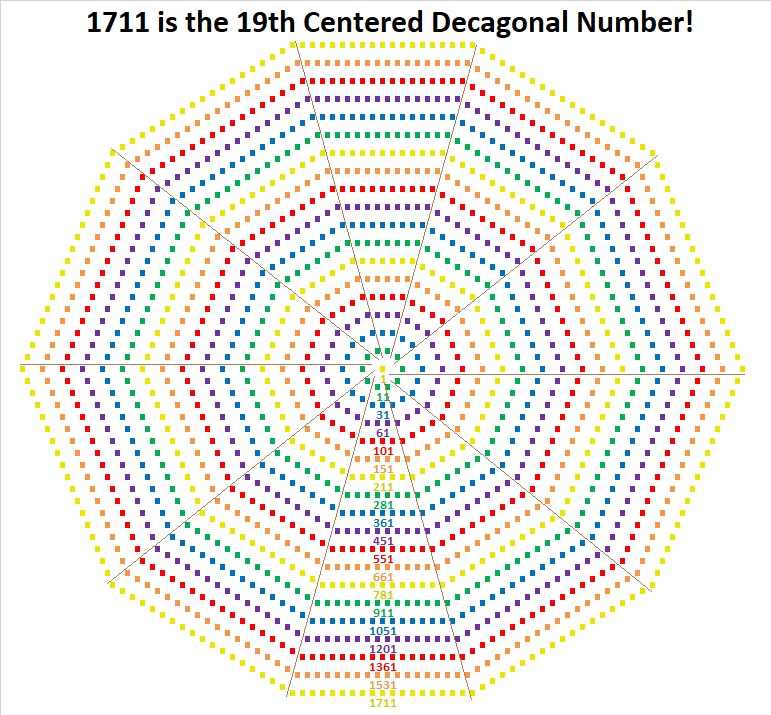Now get this: Not only is 1711 the 19th Centered Decagonal Number, but similar-looking 17111 is the 59th Centered Decagonal Number! (A mere coincidence, but the 59th is even cooler because 59·29 = 1711.)

### More About the Number 1711:

1711 is the hypotenuse of a Pythagorean triple:
1180-1239-1711, which is (20-21-29) times 59.

1711 is the difference of two squares in two different ways:
856² – 855² = 1711, and
44² – 15² = 1711.

1711 is a fun number to explore!

# Find the Factor Pairs of 1710. Which One Sums to 181? Which One Subtracts to 181?

### Today’s Puzzle:

1710 has TWELVE factor pairs. Two of those factor pairs are special. If you add up the factors in one of them, you will get 181, but get this, if you subtract the factors in the other one, you will also get 181. Find the two factor pairs that do that, and you will have essentially solved this puzzle:This Sum-Difference puzzle would be helpful if you had to factor any of these trinomials:
45x² – 181x + 38,
95x² – 181x – 18,
90x² + 181x + 19,
38x² + 181x – 45,

The trinomials are in the form ax² + bx + c, where b = 181, and a·c = ±1710. Whether the 1710 is positive or negative makes all the difference in the world when factoring them!

### Factors of 1710:

• 1710 is a composite number.
• Prime factorization: 1710 = 2 × 3 × 3 × 5 × 19, which can be written 1710 = 2 × 3² × 5 × 19.
• 1710 has at least one exponent greater than 1 in its prime factorization so √1710 can be simplified. Taking the factor pair from the factor pair table below with the largest square number factor, we get √1710 = (√9)(√190) = 3√190.
• The exponents in the prime factorization are 1, 2, 1, and 1. Adding one to each exponent and multiplying we get (1 + 1)(2 + 1)(1 + 1)(1 + 1) = 2 × 3 × 2 × 2 = 24. Therefore 1710 has exactly 24 factors.
• The factors of 1710 are outlined with their factor pair partners in the graphic below.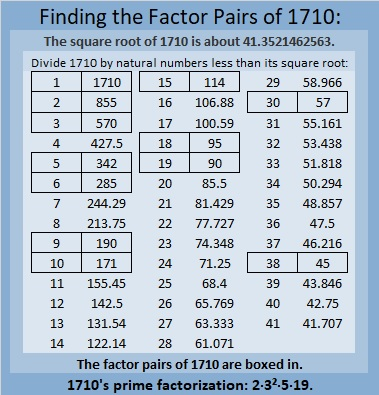The following chart lists 1710’s factor pairs with their sums and differences: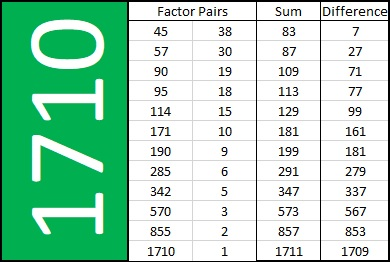### More About the Number 1710:

1710 is the hypotenuse of a Pythagorean triple:
1026-1368-1710, which is (3-4-5) times 342.

# 1709 Sometimes “Guess and Check” Is a Good Strategy

### Today’s Puzzle:

Most of the puzzles I publish are logic puzzles, and I encourage you to find the logic of the puzzle and not guess and check.  However, guess and check is a legitimate strategy in mathematics, and it is a legitimate strategy to solve this particular puzzle.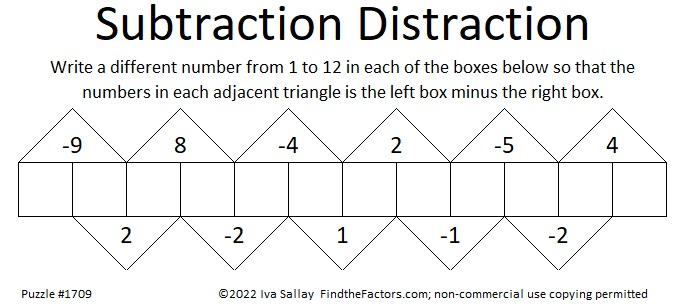Since one of the clues is -9, we know that the two boxes under it must be [1, 10], [2, 11], or [3, 12].

Suppose you assume it’s 1 – 10 = -9. If you fill out the rest of the boxes you would get: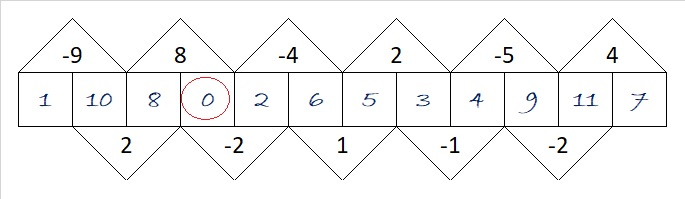You know that isn’t right because zero is not a number from 1 to 12. No problem. Simply add one to each of the numbers you wrote in, and the puzzle will be solved with only numbers from 1 to 12.

Suppose you assumed it’s 3 -12 = -9. The rest of the boxes would look like this:Again, 13 is not included in the numbers from 1 to 12, but you can fix it by subtracting 1 from each of the numbers you wrote in. Easy Peasy.

### Factors of 1709:

• 1709 is a prime number.
• Prime factorization: 1709 is prime.
• 1709 has no exponents greater than 1 in its prime factorization, so √1709 cannot be simplified.
• The exponent in the prime factorization is 1. Adding one to that exponent we get (1 + 1) = 2. Therefore 1709 has exactly 2 factors.
• The factors of 1709 are outlined with their factor pair partners in the graphic below.How do we know that 1709 is a prime number? If 1709 were not a prime number, then it would be divisible by at least one prime number less than or equal to √1709. Since 1709 cannot be divided evenly by 2, 3, 5, 7, 11, 13, 17, 19, 23, 29, 31, 37, or 41, we know that 1709 is a prime number.

### More About the Number 1709:

1709 is the sum of two squares:
35² + 22² = 1709.

1709 is the hypotenuse of a Pythagorean triple:
741-1540-1709, calculated from 35² – 22², 2(35)(22), 35² + 22².

Here’s another way we know that 1709 is a prime number: Since its last two digits divided by 4 leave a remainder of 1, and 35² + 22² = 1709 with 35 and 22 having no common prime factors, 1709 will be prime unless it is divisible by a prime number Pythagorean triple hypotenuse less than or equal to √1709. Since 1709 is not divisible by 5, 13, 17, 29, 37, or 41, we know that 1709 is a prime number.